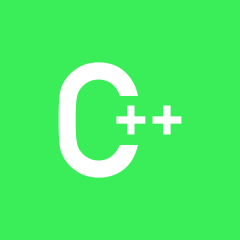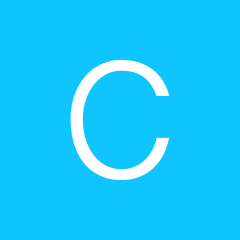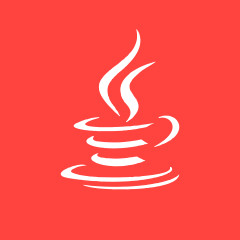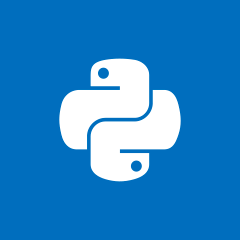C++C++ CC C++C++ JAVAC++ PHPC++ CC++ PHPC++ JAVA JQueryJAVA C++C++ CC++ CC C++ 数据结构C++
C语言编程时候的运行问题！求大佬指教！C C# C++
c++的编辑软件C++C++ CC++ CC++ CC++ PHPC++ CC++ PHPJAVA C++C++ CC++ CC++ PHPJAVA C++C++ PHPC++ CPython C++C++ PHPJAVA C++